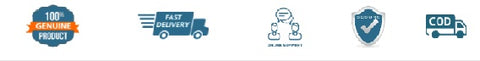# NCERT Mathematics - Class 6 - latest edition as per NCERT/CBSE

SKU:3MNCERT033
Price : Rs. 95.00
Sale price Rs. 95.00 Price : Rs. 105.00
Sold out
Unit price
per
Shipping calculated at checkout.

NCERT Mathematics - Class 6 - latest edition as per NCERT/CBSE

Chapters
• Knowing Our Numbers
• Whole Numbers
• Playing With Numbers
• Basic Geometrical Ideas
• Understanding Elementary Shapes
• Integers
• Fractions
• Decimals
• Data Handling
• Mensuration
• Algebra
• Ratio And Proportion
• Symmetry
• Practical Geometry

(All books are 100℅ original and as per the latest NCERT syllabus. Prices includes Rs. 40 per book mandatory binding charges.)